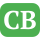CppBuzz.com

# Functions in C Programming

Functions are named section which contains group of statements to solve some problem. Functions are used to break large program into smaller ones. Functions are created when same piece of code is begin used multiple times. Functions can accepts arguments and return some value. Name of function can be anything but ideally it should be meaningful.
Note - We can't have two functions with same name in C programing.

## Syntax of function

` returntype functionname(arg1, arg2..);`

Here returntype can be anything from void to predefined data type (char, int, float, etc..) or user defined data type (structure, enum or union)

## Functions may have three sections

• Prototype / Declaration
• Function call
• Function body / Definition

## When function body is above main() function

When have funtion body (definition) on above main() function then we dont' need to declare it. Look the example, when function call occurs the code looks for the function definition. If definition is not found then we get error "functon definition not found". If defintion is found then controll jumps to definition and start execution each statements. After execution all statements the controll jump to next line of function call and if there is code after function call then that is executed.

```#include<stdio.h>

//this is function definition
void hello()
{
printf("\n Hello CppBuzz.com");
}

main()
{
hello(); //function call
}
```

## When function body is below main()

```#include<stdio.h>

//function prototype - optional
void hello();

main()
{
hello(); //function call
}

//this is function definition
void hello()
{
printf("\n Hello CppBuzz.com");
}
```

## Return value in functions

As discussed earlier, function can return any type of value. Lets see an example where function hello() would return int value i.e 0

```#include<stdio.h>

//this is function definition
int hello()
{
printf("\n Hello CppBuzz.com");
return 0;
}

main()
{
int ret;

ret = hello(); //save return value in ret variable
printf("\n return value of function is : %d", ret);
}
```

## Passing Arguments to function

```#include<stdio.h>

//function which accepts one argument of type int
void hello(int a)
{
printf("\n Hello CppBuzz.com");
printf("\n The value is a is : %d", a);
}

int main()
{
int aa = 5;
hello(aa); //passing aa as argument
return 0;
}
```

Here aa is called actual argument while a in argument of function definition is known as formal argument

## Passing Arguments and return value

```#include<stdio.h>

//this is function definition
int  intsum(int aa, int bb)
{
printf("\n Hello CppBuzz.com");

return aa+bb;
}

int main()
{
int a = 5;
int b = 10;
int sum;

sum = intsum(a, b); //passing a & b as argument

printf("\n Sum of %d & %d is %d ",a,b,sum);
return 0;
}
```

## Recursion in function

When funcion call itself it is known as recursion. To come out of recusion we have to put some condition and it is checked everytime before calling the function.

```#include<stdio.h>

void recursion(int aa)
{
static int counter = 0;
printf("%d,",aa);

if(counter<5){
counter++;
recursion(aa); //function calling itself
}

}

int main()
{
int a = 5;
recursion(a); //passing a
return 0;
}
```